Updating search results...

# 193 Results

View
Selected filters:
• FunctionsConditional Remix & Share Permitted
CC BY-NC-SA
Rating
0.0 stars

Le contenu de ce manuel couvre la grande majorité des sujets présentés dans les cours de calcul différentiel et intégral pour les étudiants en sciences et génie. Les seuls préalables sont les mathématiques normalement enseignées au secondaire en Ontario. Ce manuel peut être utilisé pour trois des variantes des cours de calcul différentiel et intégral que nous retrouvons dans la majorité des universités en Ontario : Calcul différentiel et intégral pour les étudiants en génie, Calcul différentiel et intégral pour les étudiants en sciences de la vie et Calcul différentiel et intégral pour les étudiants en administration.

Subject:
Algebra
Calculus
Functions
Mathematics
Material Type:
Textbook
Author:
Benoit Dionne
05/16/2023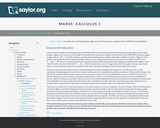Unrestricted Use
CC BY
Rating
0.0 stars

This course begins with a review of algebra specifically designed to help and prepare the student for the study of calculus, and continues with discussion of functions, graphs, limits, continuity, and derivatives. The appendix provides a large collection of reference facts, geometry, and trigonometry that will assist in solving calculus problems long after the course is over. Upon successful completion of this course, the student will be able to: calculate or estimate limits of functions given by formulas, graphs, or tables by using properties of limits and LĺÎĺ_ĺĚĺ_hopitalĺÎĺ_ĺĚĺ_s Rule; state whether a function given by a graph or formula is continuous or differentiable at a given point or on a given interval and justify the answer; calculate average and instantaneous rates of change in context, and state the meaning and units of the derivative for functions given graphically; calculate derivatives of polynomial, rational, common transcendental functions, and implicitly defined functions; apply the ideas and techniques of derivatives to solve maximum and minimum problems and related rate problems, and calculate slopes and rates for function given as parametric equations; find extreme values of modeling functions given by formulas or graphs; predict, construct, and interpret the shapes of graphs; solve equations using NewtonĺÎĺ_ĺĚĺ_s Method; find linear approximations to functions using differentials; festate in words the meanings of the solutions to applied problems, attaching the appropriate units to an answer; state which parts of a mathematical statement are assumptions, such as hypotheses, and which parts are conclusions. This free course may be completed online at any time. It has been developed through a partnership with the Washington State Board for Community and Technical Colleges; the Saylor Foundation has modified some WSBCTC materials. (Mathematics 005)

Subject:
Calculus
Functions
Mathematics
Material Type:
Assessment
Full Course
Syllabus
Textbook
Provider:
The Saylor Foundation
04/16/2012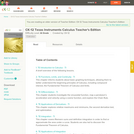Conditional Remix & Share Permitted
CC BY-NC-SA
Rating
0.0 stars

CK-12's Texas Instruments Calculus Teacher's Edition is a useful companion to a Calculus course, offering extra assignments and opportunities for students to understand course material through their graphing calculator.

Subject:
Calculus
Functions
Mathematics
Material Type:
Textbook
Provider:
CK-12 Foundation
Provider Set:
CK-12 FlexBook
Author:
Jordan, Lori
12/17/2010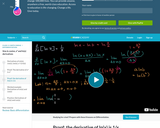Conditional Remix & Share Permitted
CC BY-NC-SA
Rating
0.0 stars

We told you about the derivatives of many functions, but you might want proof that what we told you is actually true. That's what this tutorial tries to do!

Subject:
Calculus
Functions
Mathematics
Material Type:
Lecture
Provider:
Provider Set:
05/30/2013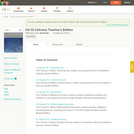Conditional Remix & Share Permitted
CC BY-NC-SA
Rating
0.0 stars

CK-12 Calculus Teacher's Edition covers tips, common errors, enrichment, differentiated instruction and problem solving for teaching CK-12 Calculus Student Edition. The solution guide is available upon request.

Subject:
Calculus
Functions
Mathematics
Material Type:
Textbook
Provider:
CK-12 Foundation
Provider Set:
CK-12 FlexBook
Author:
Dreyfuss, Andrew
Narasimhan, Ramesh
Prolo, Jared
06/24/2011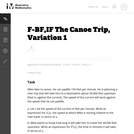Unrestricted Use
CC BY
Rating
0.0 stars

The purpose of this task is to give students practice constructing functions that represent a quantity of interest in a context, and then interpreting features of the function in the light of that context. It can be used as either an assessment or a teaching task.

Subject:
Functions
Mathematics
Material Type:
Activity/Lab
Provider:
Illustrative Mathematics
Provider Set:
Illustrative Mathematics
Author:
Illustrative Mathematics
05/01/2012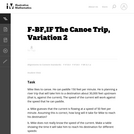Unrestricted Use
CC BY
Rating
0.0 stars

The primary purpose of this task is to lead students to a numerical and graphical understanding of the behavior of a rational function near a vertical asymptote, in terms of the expression defining the function. The canoe context focuses attention on the variables as numbers, rather than as abstract symbols.

Subject:
Functions
Mathematics
Material Type:
Activity/Lab
Provider:
Illustrative Mathematics
Provider Set:
Illustrative Mathematics
Author:
Illustrative Mathematics
05/01/2012Unrestricted Use
CC BY
Rating
0.0 stars

The task requires the student to use logarithms to solve an exponential equation in the realistic context of carbon dating, important in archaeology and geology, among other places. Students should be guided to recognize the use of the natural logarithm when the exponential function has the given base of e, as in this problem. Note that the purpose of this task is algebraic in nature -- closely related tasks exist which approach similar problems from numerical or graphical stances.

Subject:
Archaeology
Functions
Mathematics
Social Science
Material Type:
Activity/Lab
Provider:
Illustrative Mathematics
Provider Set:
Illustrative Mathematics
Author:
Illustrative Mathematics
05/01/2012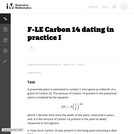Unrestricted Use
CC BY
Rating
0.0 stars

In the task "Carbon 14 Dating'' the amount of Carbon 14 in a preserved plant is studied as time passes after the plant has died. In practice, however, scientists wish to determine when the plant died and, as this task shows, this is not possible with a simple measurement of the amount of Carbon 14 remaining in the preserved plant. The equation for the amount of Carbon 14 remaining in the preserved plant is in many ways simpler here, using 12 as a base.

Subject:
Functions
Mathematics
Material Type:
Activity/Lab
Provider:
Illustrative Mathematics
Provider Set:
Illustrative Mathematics
Author:
Illustrative Mathematics
08/21/2012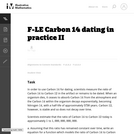Unrestricted Use
CC BY
Rating
0.0 stars

This problem introduces the method used by scientists to date certain organic material. It is based not on the amount of the Carbon 14 isotope remaining in the sample but rather on the ratio of Carbon 14 to Carbon 12. This ratio decreases, hypothetically, at a constant exponential rate as soon as the organic material has ceased to absorb Carbon 14, that is, as soon as it dies. This problem is intended for instructional purposes only. It provides an interesting and important example of mathematical modeling with an exponential function.

Subject:
Functions
Mathematics
Material Type:
Activity/Lab
Provider:
Illustrative Mathematics
Provider Set:
Illustrative Mathematics
Author:
Illustrative Mathematics
08/21/2012Unrestricted Use
CC BY
Rating
0.0 stars

This exploratory task requires the student to use a property of exponential functions in order to estimate how much Carbon 14 remains in a preserved plant after different amounts of time.

Subject:
Functions
Mathematics
Material Type:
Activity/Lab
Provider:
Illustrative Mathematics
Provider Set:
Illustrative Mathematics
Author:
Illustrative Mathematics
05/01/2012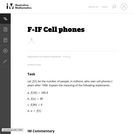Unrestricted Use
CC BY
Rating
0.0 stars

This simple task assesses whether students can interpret function notation. The four parts of the task provide a logical progression of exercises for advancing understanding of function notation and how to interpret it in terms of a given context.

Subject:
Functions
Mathematics
Material Type:
Activity/Lab
Provider:
Illustrative Mathematics
Provider Set:
Illustrative Mathematics
Author:
Illustrative Mathematics
05/01/2012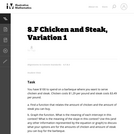Unrestricted Use
CC BY
Rating
0.0 stars

This task presents a real world situation that can be modeled with a linear function best suited for an instructional context.

Subject:
Functions
Mathematics
Material Type:
Activity/Lab
Provider:
Illustrative Mathematics
Provider Set:
Illustrative Mathematics
Author:
Illustrative Mathematics
05/01/2012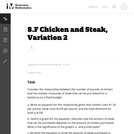Unrestricted Use
CC BY
Rating
0.0 stars

This task is intended strictly for instructional purposes with the goal of building understandings of linear relationships within a meaningful and, hopefully, somewhat familiar context.

Subject:
Functions
Mathematics
Material Type:
Activity/Lab
Provider:
Illustrative Mathematics
Provider Set:
Illustrative Mathematics
Author:
Illustrative Mathematics
05/01/2012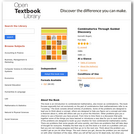Some Rights Reserved
Rating
0.0 stars

Subject:
Functions
Mathematics
Material Type:
Textbook
Provider:
Dartmouth College
Author:
Kenneth Bogart
01/01/2004Unrestricted Use
CC BY
Rating
0.0 stars

This task gives students an opportunity to work with exponential functions in a real world context involving continuously compounded interest. They will study how the base of the exponential function impacts its growth rate and use logarithms to solve exponential equations.

Subject:
Functions
Mathematics
Material Type:
Activity/Lab
Provider:
Illustrative Mathematics
Provider Set:
Illustrative Mathematics
Author:
Illustrative Mathematics
05/01/2012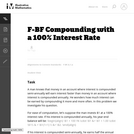Unrestricted Use
CC BY
Rating
0.0 stars

This task is preliminary to F-LE Compounding Interest with a 5% Interest Rate which further develops the relationship between e and compound interest.

Subject:
Functions
Mathematics
Material Type:
Activity/Lab
Provider:
Illustrative Mathematics
Provider Set:
Illustrative Mathematics
Author:
Illustrative Mathematics
05/01/2012Unrestricted Use
CC BY
Rating
0.0 stars

This task develops reasoning behind the general formula for balances under continuously compounded interest. While this task itself specifically addresses the standard (F-BF), building functions from a context, a auxiliary purpose is to introduce and motivate the number e, which plays a significant role in the (F-LE) domain of tasks.

Subject:
Functions
Mathematics
Material Type:
Activity/Lab
Provider:
Illustrative Mathematics
Provider Set:
Illustrative Mathematics
Author:
Illustrative Mathematics
05/01/2012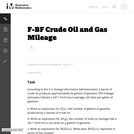Unrestricted Use
CC BY
Rating
0.0 stars

This word problem requires students to create expressions to calculate gas milage for a vehicle.

Subject:
Functions
Mathematics
Material Type:
Activity/Lab
Provider:
Illustrative Mathematics
Provider Set:
Illustrative Mathematics
Author:
Illustrative Mathematics
05/01/2012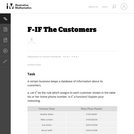Unrestricted Use
CC BY
Rating
0.0 stars

The purpose of this task is to introduce or reinforce the concept of a function, especially in a context where the function is not given by an explicit algebraic representation. Further, the last part of the task emphasizes the significance of one variable being a function of another variable in an immediately relevant real-life context.

Subject:
Functions
Mathematics
Material Type:
Activity/Lab
Provider:
Illustrative Mathematics
Provider Set:
Illustrative Mathematics
Author:
Illustrative Mathematics